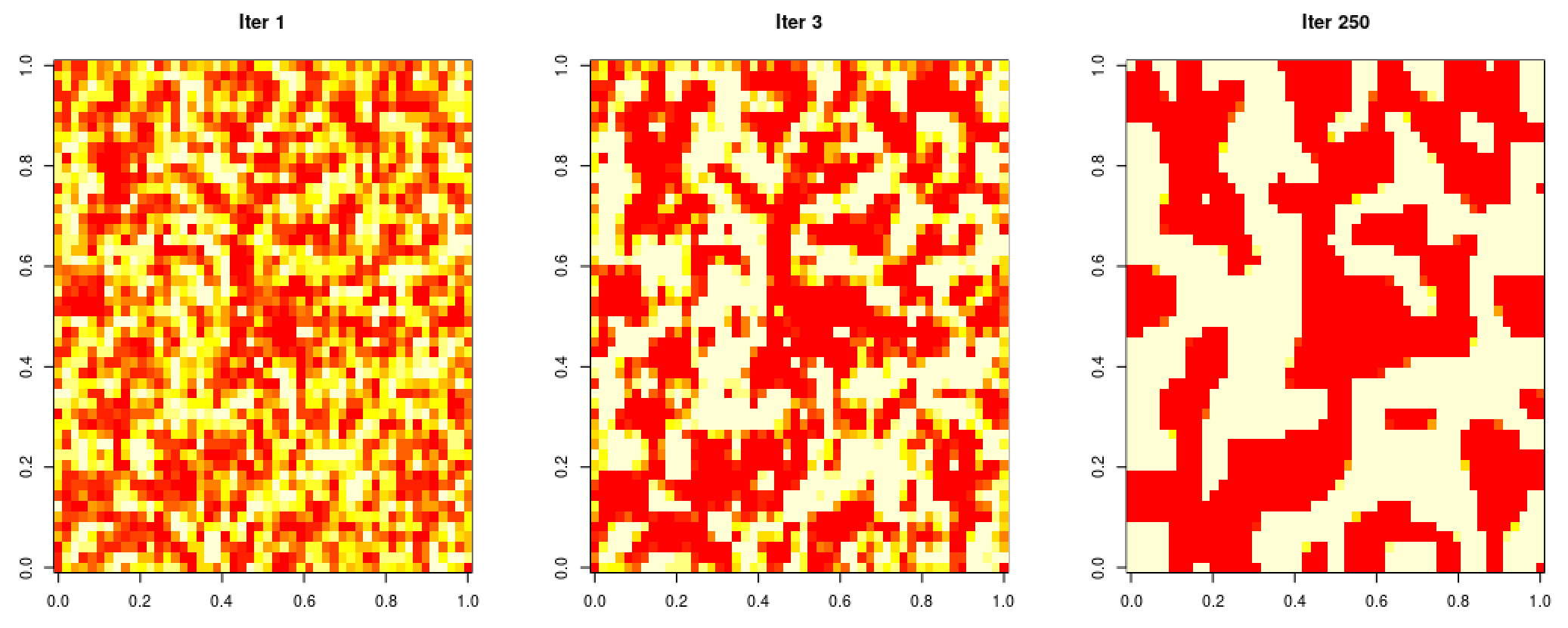## … using Stan & HMC

Here, I sample from an Ising-like model (I treat the random variables as continuous, between -1 and 1 and add a term to the pseudo-likelihood that resembles a beta log density).

The matrix terms are essentially a vectorised product-sum of nearest neighbour spins.

Results:## 2020

### Efficient Gaussian Process Computation

I’ll try to give examples of efficient gaussian process computation here, like the vec trick (Kronecker product trick), efficient toeliptz and circulant matrix computations, RTS smoothing and Kalman filtering using state space representations, and so on.

## Minimal Working Examples

### Gaussian Process Speech Synthesis (Draft)

Very untidy first working draft of the idea mentioned on the efficient computation page. Here, I fit a spectral mixture to some audio data to build a “generative model” for audio. I’ll implement efficient sampling later, and I’ll replace the arbitrary way this is trained with an LSTM-RNN to go straight from text/spectrograms to waveforms.

# Random Projects

### Gaussian Process Middle C

First of my experiments on audio modelling using gaussian processes. Here, I construct a GP that, when sampled, plays middle c the way a grand piano would.

Consider: